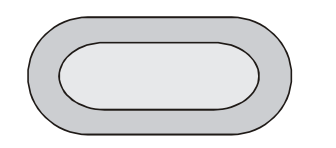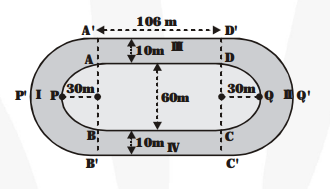# Fig. depicts a racing track whose left and right ends are semicircular.

Question:

Fig. depicts a racing track whose left and right ends are semicircular.The distance between the two inner parallel line segments is 60 m and they are each 106 m long. If the track is 10 m wide, find:

(i) the distance around the track along its inneredge

(ii) the area of the track.

Solution:

(i) The distance around the track along the inner edge (as seen from figure)= Perimeter of APB + BC + Perimeter CQD + AD

$=\{\pi \times 30+106+\pi \times 30+106\} \mathrm{m}$

$=\{60 \pi+212\} \mathrm{m}$

$=\left\{60 \times \frac{22}{7}+212\right\}=\frac{2804}{7} \mathbf{m}$

(ii) Area of region $\mathrm{I}=\frac{\mathbf{1}}{\mathbf{2}} \pi \times(40)^{2}-\frac{\mathbf{1}}{\mathbf{2}} \pi \times(30)^{2}$

$\{\because$ outer radius $=30 \mathrm{~m}+10 \mathrm{~m}=40 \mathrm{~m}\}$

$=\frac{\mathbf{1}}{\mathbf{2}} \pi \times 700 \mathrm{~m}^{2}=\frac{\mathbf{1}}{\mathbf{2}} \times \frac{\mathbf{2} 2}{\mathbf{7}} \times 700 \mathrm{~m}^{2}$

$=1100 \mathrm{~m}^{2}$

Similarly, area of the region II $=1100 \mathrm{~m}^{2}$

Area of the region III (106 m × 10 m rectangle)

$=106 \times 10=1060 \mathrm{~m}^{2}$

Similarly, the area of the region IV $=1060 \mathrm{~m}^{2}$

Then, the total area of the track

$=2 \times 1100 \mathrm{~m}^{2}+2 \times 1060 \mathrm{~m}^{2}$

$=(2200+2120) \mathrm{m}^{2}=4320 \mathrm{~m}^{2}$